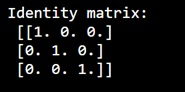# How to print a unit matrix?

Learn, how to print a unit matrix in Python?
Submitted by Pranit Sharma, on January 01, 2023

NumPy is an abbreviated form of Numerical Python. It is used for different types of scientific operations in python. Numpy is a vast library in python which is used for almost every kind of scientific or mathematical operation. It is itself an array which is a collection of various methods and functions for processing the arrays.

## Printing a unit matrix

A unit matrix is that which has only 1 element and its value is also 1.
Here, we need to print a sort of identity matrix where only one value in each row is 1 and the other elements are zero.

Suppose we need to print an identity matrix in such a way that or each row, one value should be 1, and the others 0; on the top row the left-most value should be 1; on the bottom row the right-most value should be 1; these values should form a diagonal line through the matrix.

For this purpose, we will use numpy.identity() method inside which we will pass integer values that represents the size of this matrix. An important point is that this matrix contains an equal number of rows and columns.

Let us understand with the help of an example,

## Python code to print a unit matrix

```# Import numpy
import numpy as np

# Defining the fixed values
# for size of matrix
n = 3

# Creating an identity matrix
res = np.identity(n)

# Display result
print("Identity matrix:\n",res,"\n")
```

Output: